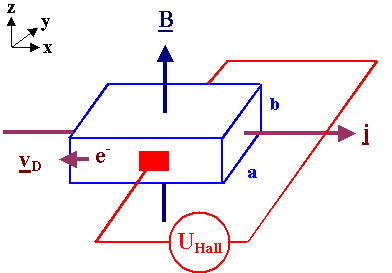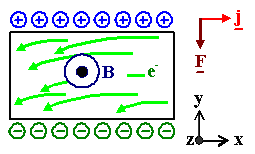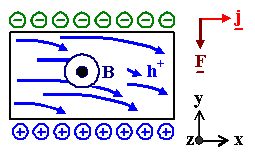### 2.1.3 The Hall EffectThis subchapter introduces two important topics: The Hall effect as an important observation in materials science and at the same time another irrefutable proof that classical physics just can't hack it when it comes to electrons in crystals.The Hall effect describes what happens to current flowing through a conducting material - a metal, a semiconductor - if it is exposed to a magnetic field B.We will look at this in classical terms; again we will encounter a fundamental problem.The standard geometry for doing an experiment in its most simple form is as follows:Relevant vectors; the current is carried by electronsA magnetic field B is employed perpendicular to the current direction j, as a consequence a potential difference (i.e. a voltage) develops at right angles to both vectors.In other words: A Hall voltage UHall will be measured perpendicular to B and j.In yet other words: An electrical field  EHall develops in y-directionThat is already the essence of the Hall effect.It is relatively easy to calculate the magnitude of the Hall voltage UHall that is induced by the magnetic field B.First we note that we must also have an electrical field E parallel to j because it is the driving force for the current.Second, we know that a magnetic field at right angles to a current causes a force on the moving carriers, the so-called Lorentz force FL, that is given by
FL  =  q · (vD × B)We have to take the drift velocity vD of the carriers, because the other velocities (and the forces caused by these componentes) cancel to zero on average. The vector product assures that FL is perpendicular to vD and B.Note that instead the usual word "electron" the neutral term carrier is used, because in principle an electrical current could also be carried by charged particles other than electrons, e.g. positively charged ions. Remember a simple but important picture given before!For the geometry above, the Lorentz force FL has only a component in y - direction and we can use a scalar equation for it. Fy is given by
Fy  =  q · vD,x · BzWe have to be a bit careful: We know that the force is in y-direction, but we do no longer know the sign. It changes if either q, vD, or Bz changes direction and we have to be aware of that.However, it is important to note that for a fixed current density jx the direction of the Lorentz force is independent of the sign of the charge carriers – the sign of the charge and the sign of the drift velocity just cancel each other.With vD = µ · E and µ = mobility of the carriers, we obtain a rather simple equation for the force
Fy  =  – |q| · µ · Ex · BzThis means that the current of carriers will be deflected from a straight line in y-direction. In other words, there is a component of the velocity in y-direction and the surfaces perpendicular to the y-direction will become charged as soon as the current (or the magnetic field) is switched on. The flow-lines of the carriers will look like this:The charging of the surfaces is unavoidable, because some of the carriers eventually will end up at the surface where they are "stuck".Notice that the sign of the charge for a given surface depends on the sign of the charge of the carriers. Negatively charged electrons (e- in the picture) end up on the surface opposite to posively charged carriers (called h+ in the picture).Notice, too, that the direction of the force Fy is the same for both types of carriers, simply because both q and vD change signs in the force formulaThe surface charge then induces an electrical field Ey in y-direction which opposes the Lorentz force; it tries to move the carriers back.In equilibrium, the Lorentz force Fy and the force from the electrical field Ey in y-direction (which is of course simply q · Ey) must be equal with opposite signs. We therefore obtain
q · Ey  =  – |q| · µ · Ex · Bz

Ey  =  sgn(q) · µ · Ex · BzThe Hall voltage UHall now is simply the field in y-direction multiplied by the dimension dy in y-direction.It is clear then that the (easily measured) Hall voltage is a direct measure of the mobility µ of the carriers involved, and that its sign or polarity will change if the sign of the charges flowing changes.It is customary to define a Hall coefficient RHall for a given material.This can be done in different, but equivalent ways. In the link we look at a definition that is particularly suited for measurements. Here we use the following definition:
RHall  =  Ey
Bz · jxIn other words, we expect that the Hall voltage Ey · dy (with dy = dimension in y-direction) is proportional to the current(density) j and the magnetic field strength B, which are, after all, the main experimental parameters (besides the trivial dimensions of the specimen):
Ey  =  RHall · Bz · jxThe Hall coefficient is a material parameter, indeed, because we will get different numbers for RHall if we do experiments with identical magnetic fields and current densities, but different materials. The Hall coefficient, as mentioned before, has interesting properties:RHall will change its sign, if the sign of the carriers is changed because then Ey changes its sign, too. It thus indicates in the most unambiguous way imaginable if positive or negative charges carry the current.RHall allows to obtain the mobility µ of the carriers, too, as we will see immediately.RHall is easily calculated: Using the equation for Ey from above, and the basic equation  jx = s · Ex, we obtain for negatively charged carriers:
RHall   =  –   µ · Ex · Bz
s · Ex · Bz
=  –   µ
s
=   µ
|q| · n · µ
=   – 1
|q| · nThe blue part corresponds to the derivation given in the link; n is (obviously) the carrier concentration.If one knows the Hall coefficient or the carrier concentration, the Hall effect can be used to measure magnetic field strengths B ( not so easily done otherwise!).Measurements of the Hall coefficient of materials with a known conductivity (something easily measurable) thus give us directly the mobility of the carriers responsible for the conductance.The minus sign above is obtained for electrons, i.e. negative charges.If positively charged carriers would be involved, the Hall constant would be positive.Note that while it is not always easy to measure the numerical value of the Hall voltage and thus of R with good precision, it is the easiest thing in the world to measure the polarity of a voltage.Let's look at a few experimental data:
 Material Li Cu Ag Au Al Be In Semiconductors (e.g. Si, Ge, GaAs, InP,...) R (× 10–24) cgs units –1,89 –0,6 –1,0 –0,8 +1,136 +2,7 +1,774 positive or negative values, depending on "doping" Comments: 1. the positive values for the metals were measured under somewhat special conditions (low temperatures; single crystals with special orientations), for other conditions negative values can be obtained, too. 2. The units are not important in the case, but multiplying with  9 · 1013 yields the value in m3/CoulombWhichever way we look at this, one conclusion is unavoidable:In certain materials including metals, the particles carrying the electrical current are positively charged under certain conditions. And this is positively not possible in a classical model that knows only negatively charged electrons as carriers of electrical current in solids!Again we are forced to conclude:
 There is no way to describe conductivity in metals and semiconductors with classical physics!
 Fragebogen / Questionaire Multiple Choice Fragen zu 2.1.3

© H. Föll (MaWi 2 Skript)